Kain, G., Barbu, M. C., Hinterreiter, S., Richter, K., and Petutschnigg, A. (2013). "Using bark as a heat insulation material," BioRes. 8(3), 3718-3731.

#### Abstract

Spruce bark particles were used as an insulation fill material for the thermal insulation of a timber frame wall which was subjected to a simulated winter temperature difference between indoor and outdoor climate. The temperature profile development of the wall’s cross section was modeled using Fourier’s transient heat flow theory. It was shown that bark layers conducted heat more slowly than commonly known blow-in insulation materials because of their low thermal diffusivity. Moreover, material moisture development due to water vapor streams caused by vapor pressure differences between the inside and outside climate was studied, and it supported general timber construction rules.

Using Bark as a Heat Insulation Material

Günther Kain,a,* Marius-Catalin Barbu,a,b Stefan Hinterreiter,a Klaus Richter,c and Alexander Petutschnigg a

Spruce bark particles were used as an insulation fill material for the thermal insulation of a timber frame wall which was subjected to a simulated winter temperature difference between indoor and outdoor climate. The temperature profile development of the wall’s cross section was modeled using Fourier’s transient heat flow theory. It was shown that bark layers conducted heat more slowly than commonly known blow-in insulation materials because of their low thermal diffusivity. Moreover, material moisture development due to water vapor streams caused by vapor pressure differences between the inside and outside climate was studied, and it supported general timber construction rules.

Keywords: Tree bark; Loose bulk; Thermal conductivity; Thermal diffusivity

Contact information: a: Department of Forest Products Technology and Timber Construction, University of Applied Sciences Salzburg, Markt 136a, 5431 Kuchl, Austria; b: Faculty for Wood Engineering, University “Transilvania” Brasov, Str. Universitatii 1, 500068 Brasov, Romania; c: Department of Life Science Engineering, Center of Life and Food Sciences Weihenstephan, Technical University Munich, Winzererstraße 45, 80797 Munich, Germany;

* Corresponding author: gkain.lba@fh-salzburg.ac.at

INTRODUCTION

The resource availability of insulation materials made out of renewable materials is not promising for the coming years (Schwarzbauer 2005). With regard to the scarce resource availability in Central Europe, the development of new raw material sources has become increasingly important (Barbu 2011).

Because 40% of the entire European energy consumption is caused by buildings, the European Union has passed a new directive concerning the total energy efficiency of buildings. From 2018, for public buildings, and 2020, for private housing, the energy consumption of new buildings has to be significantly reduced (European Union 2010). This will create an extensive demand for efficiently insulated wall and roof systems.

With regard to life-cycle considerations, insulation materials that have low CO2 emissions both in production and in disposal at the end of the life cycle are preferred.

Ligno-cellulosic materials are very advantageous in that respect because their biological production in the photosynthesis process is based on the uptake of high amounts of COfrom the atmosphere. For the production of 1000 kg of wood, roughly 1855 kg of COare absorbed and reduced to carbon, which is directly incorporated into the biopolymers constituting the cell walls of the lignofibers. Based on the material production level, all ligno-cellulosic materials have a neutral CO2-balance (Wegener and Zimmer 1997).

The average bark content of a tree is approximately 10% of the total stem volume, and the global logging harvest for industrial purposes is roughly 1.6 billion solid m³, which results in a bark volume of 160 million m³ worldwide annually (Xing et al. 2006). Bark is the peripheral protective tissue of a tree which protects it from physical and biological exterior attacks. Therefore, it is equipped with interesting properties such as low density, low thermal conductivity, fire resistance and high fungi resistance (Fengel and Wegener 2003).

Although bark is available in large quantities and has good physical properties, it is normally not used for high value added products (e.g., particleboard production (Gupta et al. 2011; Kraft 2007)), yet combustion for thermal energy generation is the major industrial use. Recently Kain et al. (2013) analysed the suitability of bark application for insulation material use. They pressed light bark-based insulation panels with a density of 350 kg/m³ and determined a thermal conductivity of roughly 0.06 W/(m*K). It was shown that due to a relatively high density of 250 kg/m³ for bark loose bulks, the board density can’t be arbitrarily reduced and therefore the thermal conductivity was not as low as it is with the very light insulation materials (e.g., polystyrene, mineral wool, etc.), but due to the bark’s high specific heat storage capacity the thermal diffusivity following formula (5) was remarkably low, which is what makes bark insulation layers especially suitable for active heat storage insulation layers.

The existing calculation models for heat- and moisture-related building physics processes show satisfactory results (e.g., Suleiman et al. (1999)), nevertheless there is a lack of specific values for new building materials (in the present case the bark loose bulks) or at least they have not been verified.

The goal of this study was to evaluate whether bark particles could be also used in loose bulks for cavity insulation purposes and to also test whether laboratory measurements can be verified on a larger scale in order to confirm existing calculation models. Moreover, a large-scale experiment outlines how a bark insulation layer performs under winter temperature differences between inside and outside and how quickly heat is conducted.

EXPERIMENTAL

Material for the Investigation

The bark for the current study was collected in a small softwood sawmill in Salzburg, Austria. The wood species was spruce (Picea abies).

Sample taking was carried out in autumn 2012 following the method developed by Paper Wood Austria (2009) for industrial wood chips acceptance. Thereby bark chips were taken from the upper layer of the bark pile at several spots. Additionally, the bark chips were collected at an approximate depth of 30 cm to avoid changing effects at the peripheral layer. The bark was subsequently dried with a vacuum dryer from an initial moisture content of 100% to a final moisture content of 5.8%. Then, the bark material was chipped in a 4-spindle shredder with a sieve limiting the maximum particle size to 30 mm (Fig. 1). Afterwards the milled material was sieved again to remove dust and rather small particles resulting in particles with target dimensions larger than 8 mm and smaller than 30 mm. Due to the relatively coarse particles, it was anticipated that the wall filling would be a rather porous structure with holes included. Small air holes within a structure have a low thermal conductivity, reducing the average conductivity for the whole construction.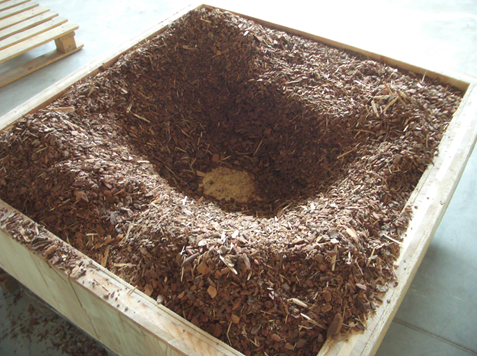Fig. 1. Bark fill material for the wall construction (particle size 8–30 mm)

Experimental Wall

To evaluate the thermal properties of a bark layer, an experimental wall was built with a length of 1300 mm, a width of 1250 mm, and a thickness of 330 mm. The framing was built out of solid pine wood with a thickness of 50 mm, which was planked on both sides with a 15-mm OSB panel. Then, temperature sensors were positioned in the center of the structure, as illustrated in Fig. 2. Afterwards, the excavation in the structure was entirely filled with the bark particles, described above, by loose pouring.

The moisture content of bark particles was determined using gravimetric moisture analysis.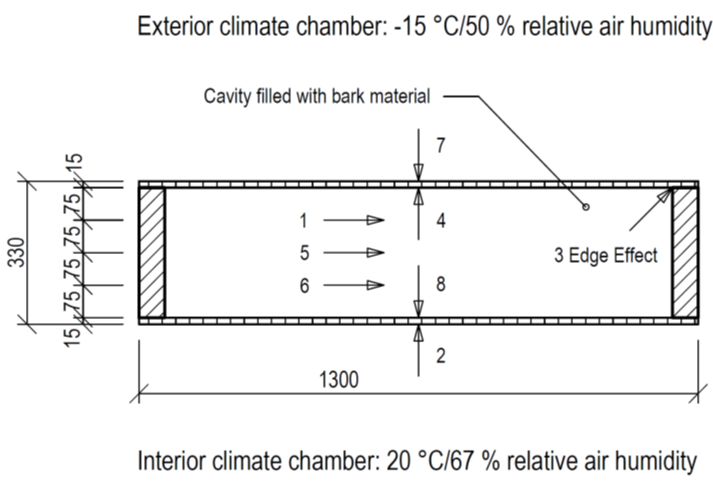Fig. 2. Sketch of the experimental wall (numbers indicate sensors)

Heat Flux Theory

Heat always flows along a temperature gradient from cold to warm, and it is stronger when that gradient is steep. For uni-dimensional heat flow, such as at a vertical wall from the inside to the outside, the model can be described as follows:

The quantity q is the heat flow density in J/(s*m²), whereas λ is the material dependent thermal conductivity according to Fourier’s first law (1). Considering that the heat quantity Q is c*ρ*V*T and its change is defined by formula (2), one gets Q/(c*ρ*V), and the temperature change is, therefore, determined by the equation in formula (3). Including formula (1) leads to Fourier’s second law for transient heat flow (in this case uni-dimensional) (4). The parameter a (5) is called “thermal diffusivity” and describes the rate at which a heat wave flows through a material (Ashby 2011; Meschede 2010).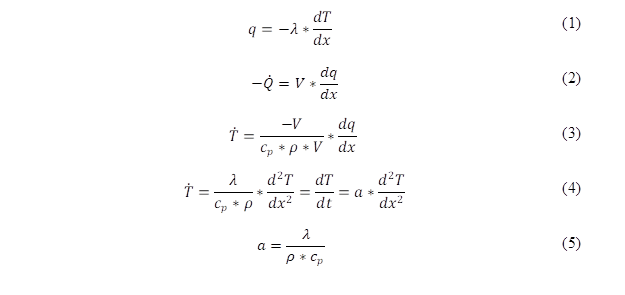Quantities in the equations can be defined as follows: q is the heat flow density in (W/m²), is the thermal conductivity (W/(m*K)), T is the absolute temperature (K), x is the horizontal position within a wall (m), t is time (s), a is thermal diffusivity (m²/s), is density (kg/m³), cp is the specific heat capacity (J/(kg*K)), w is wall thickness (m), and V is volume (m³).

Thermal Model Building

Transient heat flow problems can be solved with Fourier’s second law (4). Solutions exist for a number of standard geometries. Those can be used for a wide range of real problems which can be approximated (Ashby 2011).

The situation of the “bark wall” is approximated with the model of two semi-infinite blocks with a starting temperature of T0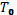and T1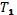brought together at time t=0 (formula (6) and Fig. 3). One assumes, therefore, that the thermal diffusivity, a, is not a function of the wall position x. The constants A and B can be defined by considering thatT0 = −15 °C andT1 = 20 °C at time t=0 in the experimental situation, with A = −T0+T1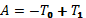and B = 2*T0T1 (OSB planking was not considered in the thermal model because of its thinness). Those considerations led to the model presented in formula (6) where “erf” is the Gauss error function.

The density of the loose bark bulk of the described fraction was previously studied by the authors (Kain et al. 2012), measured at 213 kg/m³ (standard deviation 4.0 kg/m³) when poured loosely and 258 kg/m³ (standard deviation 5.8 kg/m³) when densified through vibration. The thermal conductivity for a bark loose bulk of that density range lies between 0.057 and 0.062 W/(m*K), following a regression model calculated by the authors (Kain et al. 2013) (7). The heat storage capacity of bark was thoroughly studied by Martin (1963) who found that it is primarily influenced by the moisture and heat content of the bark. The specific heat storage capacity can be estimated by means of formula (8). That results in thermal diffusivity values for the current investigation from 1475 (−15 °C / 12% bark m.c.) to 2139 (20 °C / 29% bark m.c.) J/(kg*K).

The thermal diffusivity (5), considering the values outlined above, for bark lies between 1.027*10−7 and 1.983*10−7 m²/s. That range was taken into consideration by showing a lower and upper limit in the thermal model.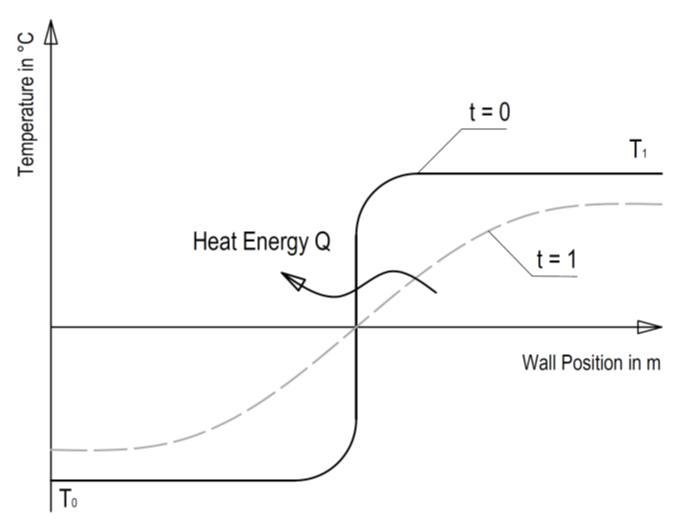Fig. 3. Sketch of the thermal model (T0 = temperature on the outer side of a construction, T1 = temperature on the inner side of a construction, “t = 0” = starting time when a temperature difference occurs, “t = 1” = some time from t0 onwards)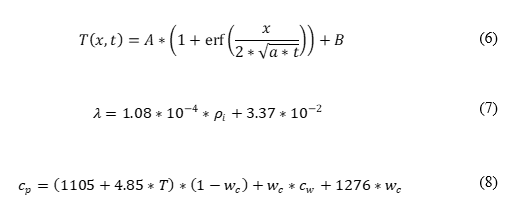whereby cp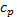is the specific heat storage capacity in J/(kg*K), T the temperature in °C, wc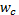the water content in kg/kg, and cw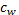the specific heat storage capacity of water which is 4185 J/(kg*K).

Water Vapor Diffusion Processes

Convection is the transport of water vapor by streaming air which can be reduced by air-proof constructions. Nevertheless, humidity can be transported as gas. As a gas, the atoms are in constant movement with an average speed of zero as their motions are oriented equally in all directions. If the atom concentration is different at two positions, atoms will flow in the direction of the lower concentration. The mass flow density, ġ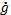, is the mass which is transported through a unit area per unit time. It is approximately proportional to the steam pressure decline (9) (Meschede 2010). The water vapor diffusion process in porous structures is hindered by the solid structure skeleton. That resistance is measured by the water vapor diffusion resistance μ (10). For the present investigation of loose bark bulk layers, a relative resistance of 5, similar to wood fibre panels, was used.In equations (9) and (10), ġ is the mass flow density in (kg/(m²*s)), δp0 is the permeability of water vapor in air (kg/(m*s*Pa)), δp is the material permeability (kg/(m*s*Pa)), dp/dx is the change of vapor pressure with position (Pa/m), and µ is the water vapor diffusion resistance.

To evaluate the vapor diffusion processes in the wall, the Glaser method was used. Therefore, the temperatures ϑl  at the material borderlines (material layers 1 to n) were calculated following formulas (11) and (12). For each temperature ϑl , the associated saturation vapor pressure was determined according to formula (13). The water vapor permeability of air for common pressures and temperatures can be estimated by δp0 ≈ (1.5*106)-1 kg/(m*h*Pa) and, therefore, the conducting resistance of a material layer can be calculated following formula (14). Partial vapor pressures at a specific wall layer can be estimated by formula (15) (Riccabona and Bednar 2010). The partial water vapor pressure in the construction layers is drawn against the saturation water vapor pressure in the Glaser diagram, and where the partial pressure is as high as the saturation pressure the condensation of water occurs.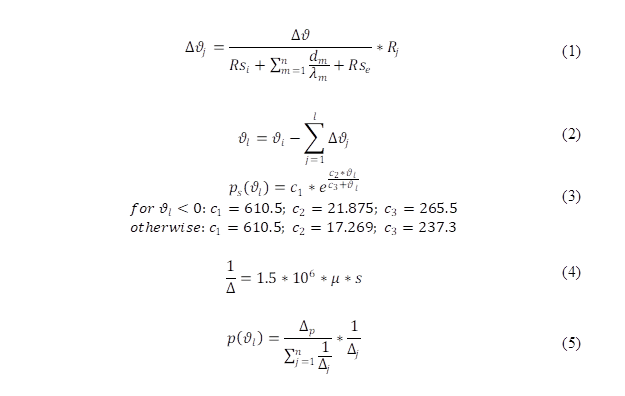In equations (9) through (15), Δϑj  is the temperature difference between the inner and outer side of layer (K), Δϑ is the temperature difference between the internal and external temperature (K), Rsi/e is the internal and accordingly external heat transmission resistance (m²*K/W), Rj  is the heat transfer resistance of layer j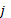(m²*K/W), ϑl is the inner surface temperature of layer l (K), ps(ϑl) is the saturation vapor pressure for ϑl (Pa),  1/Δ is the water vapor conduction resistance for a layer of thickness s (m²*h*Pa/kg), p(ϑl) is the partial vapor pressure for ϑl (Pa), and Δp is the vapor pressure difference between internal and external air (Pa).

Testing Climate

The wall described above was positioned between two climate chambers, where one simulated a climate of +20 °C and 67% relative air humidity (interior climate) and the other one −15 °C and 50% relative air humidity (exterior climate). Moisture and temperature data were recorded at different positions in the test wall in time intervals of 5 min (Fig. 2).

RESULTS AND DISCUSSION

Figures 4, 5, and 6 show the measured temperatures for sensors 1 to 8 (without edge effect 3) over the wall cross section (solid lines). Also, the calculated temperature profile according to the model (6) is shown. The parameters are the following: λ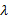= 0.062 W/(m*K), = 212 kg/m³,cp = 1475 J/(kg*K) for the low model; and λ = 0.056 W/(m*K),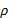= 255 kg/m³, cp = 2139 J/(kg*K) for the high model. At the beginning, the deviation between the real temperature profile and the modeled one was due to acclimatization effects as the wall was stored at a higher temperature than the chamber temperature of 20 °C (Fig. 4).

After 13 h the chamber conditions were stable and the model fit the real circumstances quite well. The temperature was only slightly underrated in the center section. After 25 h a more or less linear temperature profile over the cross section was attained where the validity of the model described ends. In that case, the fitting of the model towards real conditions is obviously best.